# Handling Vectors Specified in the i-j form

Go back to  'Vectors'

Using the $$\widehat i$$- $$\widehat j$$system, it is very easy to add or subtract vectors. Consider the following two vectors:

$\begin{array}{l}\overrightarrow {{r_1}} = 3\widehat i - 5\widehat j\\\overrightarrow {{r_2}} = - 2\widehat i + \widehat j\end{array}$

What will be the sum of these two vectors? To calculate the sum, we can simply add the $$\widehat i$$components separately, and the $$\widehat j$$components separately. Thus,

$\begin{array}{l}\overrightarrow {{r_1}} + \overrightarrow {{r_2}} = \left( {3 + \left( { - 2} \right)} \right)\widehat i + \left( {\left( { - 5} \right) + 1} \right)\widehat j\\\,\,\,\,\,\,\,\,\,\,\,\,\,\quad = \widehat i - 4\widehat j\end{array}$

The correctness of this answer can be verified through the following figure: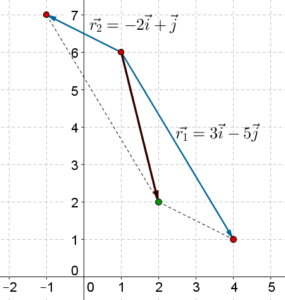Why does this method of separately adding components work? Think in terms of net effects. Suppose that

$\begin{array}{l}\overrightarrow {{r_1}} = {x_1}\widehat i + {y_1}\widehat j\\\overrightarrow {{r_2}} = {x_2}\widehat i + {y_2}\widehat j\end{array}$

Now, $$\overrightarrow {{r_1}} + \overrightarrow {{r_2}}$$ represents the net effect of $$\overrightarrow {{r_1}}$$ and $$\overrightarrow {{r_2}}$$. How can we calculate this effect?

Starting from any point, say, the origin, $$\overrightarrow {{r_1}}$$ will take us $${x_1}$$ units in the $$\widehat i$$-direction and $${y_1}$$ units in the $$\widehat j$$-direction: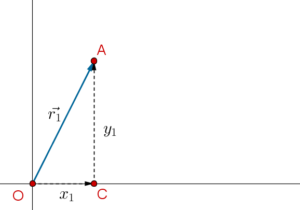Now, if we apply a displacement of $$\overrightarrow {{r_2}}$$, this will take us another $${x_2}$$ units in the $$\widehat i$$-direction, and $${y_2}$$ units in the $$\widehat j$$-direction: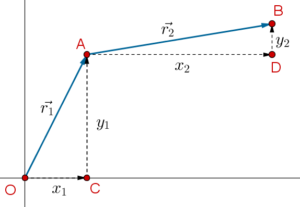If we now see our net movement in the $$\widehat i$$-direction, it is $$\left( {{x_1} + {x_2}} \right)$$, while our net movement in the $$\widehat j$$-direction is $$\left( {{y_1} + {y_2}} \right)$$. Thus, the net effect of $$\overrightarrow {{r_1}} + \overrightarrow {{r_2}}$$ was to move us $$\left( {{x_1} + {x_2}} \right)$$ units in the $$\widehat i$$-direction and $$\left( {{y_1} + {y_2}} \right)$$ units in the $$\widehat j$$-direction. This means that

$\overrightarrow {{r_1}} + \overrightarrow {{r_2}} = \left( {{x_1} + {x_2}} \right)\widehat i + \left( {{y_1} + {y_2}} \right)\widehat j$

Subtraction works similarly. Suppose that

$\overrightarrow {{r_1}} - \overrightarrow {{r_2}} = \left( {{x_1} - {x_2}} \right)\widehat i + \left( {{y_1} - {y_2}} \right)\widehat j$

The $$\widehat i$$-components and the $$\widehat j$$-components can be added separately. Verify this graphically using the following two vectors once again:

$\begin{array}{l}\overrightarrow {{r_1}} = 3\widehat i - 5\widehat j\\\overrightarrow {{r_2}} = - 2\widehat i + \widehat j\end{array}$

Example 1: Let $$\overrightarrow a$$ and $$\overrightarrow b$$ be two vectors such that

$\begin{array}{l}\overrightarrow a = 4\widehat i - 3\widehat j\\\overrightarrow b = - 2\widehat i + 7\widehat j\end{array}$

Find the magnitude of $$\left| {\overrightarrow a + \overrightarrow b } \right|$$ and $$\left| {\overrightarrow a - \overrightarrow b } \right|$$.

Solution: We have:

\begin{align}&\overrightarrow a + \overrightarrow b = \left( {4\widehat i - 3\widehat j} \right) + \left( { - 2\widehat i + 7\widehat j} \right)\\\,\,\,\,\,\,\,\,\,\,\, &\qquad\;\;\;\;= \left( {4 + \left( { - 2} \right)} \right)\widehat i + \left( { - 3 + 7} \right)\widehat j\\\,\,\,\,\,\,\,\,\,\,\, &\qquad\;\;\;\;= 2\widehat i + 4\widehat j\\&\overrightarrow a - \overrightarrow b = \left( {4\widehat i - 3\widehat j} \right) - \left( { - 2\widehat i + 7\widehat j} \right)\\\,\,\,\,\,\,\,\,\,\,\, &\qquad\;\;\;\;= \left( {4 - \left( { - 2} \right)} \right)\widehat i + \left( { - 3 - 7} \right)\widehat j\\\,\,\,\,\,\,\,\,\,\,\, &\qquad\;\;\;\;= 6\widehat i - 10\widehat j & \end{align}

Thus,

$\begin{array}{l}\left| {\overrightarrow a + \overrightarrow b } \right| = \sqrt {{2^2} + {4^2}} = \sqrt {20} = 2\sqrt 5 \\\left| {\overrightarrow a - \overrightarrow b } \right| = \sqrt {{6^2} + {{\left( { - 10} \right)}^2}} = \sqrt {136} = 4\sqrt {34} \end{array}$

Example 2: A vector $$\overrightarrow a$$ of length 5 units is inclined at an angle of 300 to the horizontal. Another vector $$\overrightarrow b$$ of length 8 units is inclined at an angle of 1200 to the horizontal: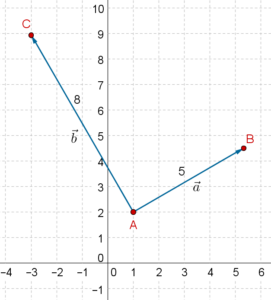Find the following vectors:

1.  $$\overrightarrow a + \overrightarrow b$$
2. $$\overrightarrow a - \overrightarrow b$$
3. $$2\overrightarrow a + 3\overrightarrow b$$

Solution: Let us first specify $$\overrightarrow a$$ and $$\overrightarrow b$$ in the $$\widehat i$$- $$\widehat j$$ system. Consider the following figure: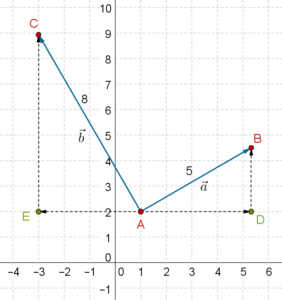We have:

$\begin{array}{l}\overrightarrow a = \overrightarrow {AD} + \overrightarrow {DB} = \left( {5\cos {{30}^0}} \right)\widehat i + \left( {5\sin {{30}^0}} \right)\widehat j\\ \qquad\qquad\quad \,\,\,\,\,\,\,\,\,\,\,\,\, = \frac{{5\sqrt 3 }}{2}\widehat i + \frac{5}{2}\widehat j\\\overrightarrow b = \overrightarrow {AE} + \overrightarrow {EC} = - \left( {8\cos {{60}^0}} \right)\widehat i + \left( {8\sin {{60}^0}} \right)\widehat j\\ \qquad\qquad\quad \,\,\,\,\,\,\,\,\,\,\,\,\, = - 4\widehat i + 4\sqrt 3 \widehat j\end{array}$

Thus,

$\begin{array}{l}\overrightarrow a + \overrightarrow b = \left( {\frac{{5\sqrt 3 }}{2} - 4} \right)\widehat i + \left( {\frac{5}{2} + 4\sqrt 3 } \right)\widehat j\\\overrightarrow a - \overrightarrow b = \left( {\frac{{5\sqrt 3 }}{2} + 4} \right)\widehat i + \left( {\frac{5}{2} - 4\sqrt 3 } \right)\widehat j\\2\overrightarrow a + 3\overrightarrow b = \left( {5\sqrt 3 \widehat i + 5\widehat j} \right) + \left( { - 12\widehat i + 12\sqrt 3 \widehat j} \right)\\\,\,\,\,\,\,\,\,\,\,\,\,\,\,\,\, = \left( {5\sqrt 3 - 12} \right)\widehat i + \left( {5 + 12\sqrt 3 } \right)\widehat j\end{array}$

Example 3: A boy is pulling a box with a force of 10 N towards the east direction. Another boy is pulling the same box with a force of 8 N at an angle of 600 to the east: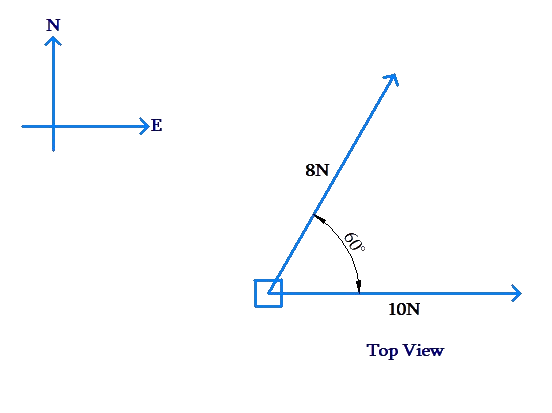If the mass of the box is 4 kg, find its acceleration $$\overrightarrow a$$.

Solution: Note that our answer should be a vector, as the acceleration of the box will have a magnitude as well as a direction. Let us establish an $$\widehat i$$- $$\widehat j$$ system such that the $$\widehat i$$-direction is the east direction, while the $$\widehat j$$-direction is the north direction: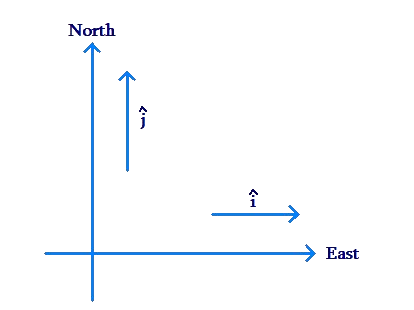Now, if the two forces being applied are represented as $$\overrightarrow {{F_1}}$$ and $$\overrightarrow {{F_2}}$$, we have:

$\begin{array}{l}\overrightarrow {{F_1}} = 10\widehat i\,\,\,{\rm{N}}\\\overrightarrow {{F_2}} = \left( {8\cos {{60}^0}} \right)\widehat i + \left( {8\sin {{60}^0}} \right)\widehat j\\\,\,\,\,\,\, = 4\widehat i + 4\sqrt 3 \widehat j\,\,\,{\rm{N}}\end{array}$

Thus,

$\overrightarrow {{F_1}} + \overrightarrow {{F_2}} = 14\widehat i + 4\sqrt 3 \widehat j\,\,\,{\rm{N}}$

Clearly, the magnitude of the resultant force is

$\left| {\overrightarrow {{F_1}} + \overrightarrow {{F_2}} } \right| = \sqrt {{{14}^2} + {{\left( {4\sqrt 3 } \right)}^2}} = \sqrt {244} \approx 15.6\,\,{\rm{N}}$

Let $$\theta$$ be the inclination of this force with the $$\widehat i$$-direction: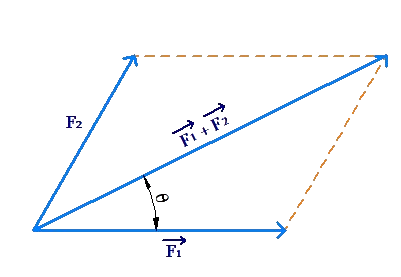Using the component form of the resultant vector, we can directly write:

\begin{align}&\tan \theta = \frac{{4\sqrt 3 }}{{14}} \approx 0.5\\&\Rightarrow \,\,\,\theta \approx {\tan ^{ - 1}}\left( {0.5} \right) \approx {26.5^0} \end{align}

The acceleration can be written as

\begin{align}&\overrightarrow a = \frac{{\overrightarrow {{F_1}} + \overrightarrow {{F_2}} }}{m} = \frac{{14\widehat i + 4\sqrt 3 \widehat j\,\,\,{\rm{N}}}}{{4\,{\rm{kg}}}}\\ \qquad\quad\;\;\; \,\,\,\,\,\,\,\,\, &\;\;\;\;= 3.5\widehat i + \sqrt 3 \widehat j\,\,\,{\rm{m/}}{{\rm{s}}^2}\end{align}

The magnitude of the acceleration is

$\left| {\overrightarrow a } \right| = \frac{{\left| {\overrightarrow {{F_1}} + \overrightarrow {{F_2}} } \right|}}{m} \approx \frac{{15.6\,\,\,{\rm{N}}}}{{4\,{\rm{kg}}}} \approx 3.9\,{\rm{m/}}{{\rm{s}}^2}$

The direction of the acceleration is the same as the direction of the net force.

Example 4: Three forces act on a box, as shown in the following figure: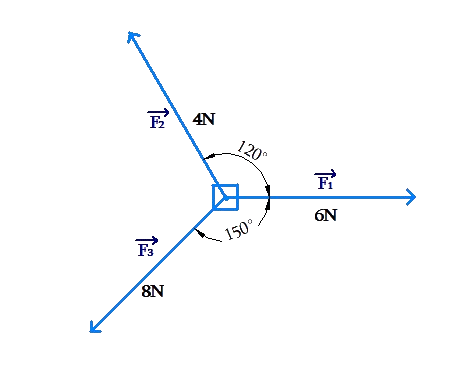Find the resultant force.

Solution: We take the direction of the first force as the $$\widehat i$$-direction, and the direction perpendicular to it as the $$\widehat j\,$$-direction: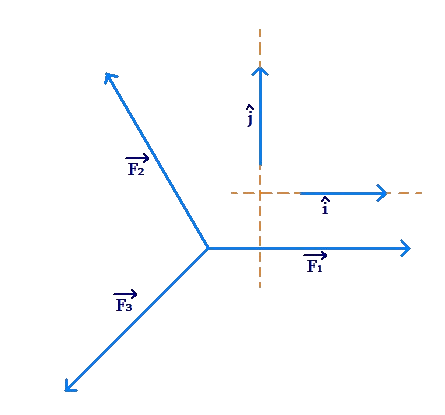Now, we specify the three forces in this $$\widehat i\, - \,\widehat j\,$$ system (the units are Newtons):

$\begin{array}{l}\overrightarrow {{F_1}} = 6\widehat i\\\overrightarrow {{F_2}} = \left( {4\cos {{120}^0}} \right)\widehat i + \left( {4\sin {{120}^0}} \right)\widehat j\,\\\,\,\,\,\,\, \;= - 2\widehat i + 2\sqrt 3 \widehat j\,\\\overrightarrow {{F_3}} = \left( {8\cos \left( { - {{150}^0}} \right)} \right)\widehat i + \left( {8\sin \left( { - {{150}^0}} \right)} \right)\widehat j\,\\\,\,\,\,\,\, \;= - 4\sqrt 3 \widehat i - 4\widehat j\,\end{array}$

The net force will be

\begin{align}&\overrightarrow {{F_{net}}} = \overrightarrow {{F_1}} + \overrightarrow {{F_2}} + \overrightarrow {{F_3}} \\\,\,\,\,\,\,\,\,\, &\quad\;\;= \left( {6\widehat i} \right) + \left( { - 2\widehat i + 2\sqrt 3 \widehat j} \right) + \left( { - 4\sqrt 3 \widehat i - 4\widehat j} \right)\\\,\,\,\,\,\,\,\,\, &\;\;\;\;\;\;= \left( {4 - 4\sqrt 3 } \right)\widehat i + \left( {2\sqrt 3 - 4} \right)\widehat j\\\,\,\,\,\,\,\,\,\, &\qquad\approx - 2.93\widehat i - 0.54\widehat j\end{align}

The magnitude of the net force is

$\left| {\overrightarrow {{F_{net}}} } \right| \approx \sqrt {{{\left( { - 2.93} \right)}^2} + {{\left( { - 0.54} \right)}^2}} \approx 2.98\,{\rm{N}}$

If $$\theta$$ is the angle of inclination of the net force with the $$\widehat i$$-direction, we have:

$\theta = - \left( {{{180}^0} - {{\tan }^{ - 1}}\left( {\frac{{0.54}}{{2.93}}} \right)} \right) \approx - {169.5^0}$

Make sure you understand this last step carefully by drawing an accurate diagram for the net force.

Vectors
grade 10 | Questions Set 1
Vectors
Vectors
grade 10 | Questions Set 2
Vectors